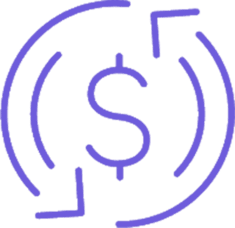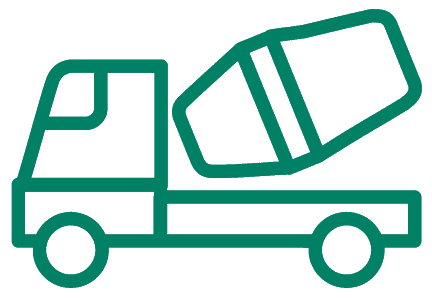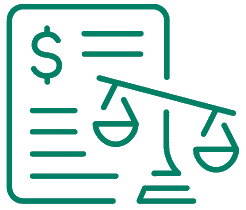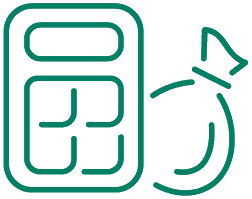## Factor rates and APR explained

Interest is generally the main cost of a business loan on top of repaying the amount borrowed (the principal).

In Australia, most loans are advertised with a fixed or variable interest rate. This 'advertised rate' only refers to the interest charges on the loan, and does not factor in any fees which may increase the total cost of the loan.

The true cost of the loan is represented by what's called the annual percentage rate (APR). This the cost of the loan per year, with application and ongoing fees take into account, expressed as a percentage of the loan balance.

The APR is therefore more beneficial, making it easier to compare multiple loan offers based on their true, total cost.

However, business loan lenders in Australia may occasionally use a factor rate in place of an interest rate, which can be confusing to borrowers who may only be familiar with traditional interest rates.

Factor rates are common when advertising business loans with terms of less than 12 months.

To understand the true cost of a business loan when only a factor rate is provided, borrowers will need to convert the factor rate to the Annual Percentage Rate (APR), which displays the interest rate as it would be if charged over a 12-month period.

## How do factor rates work?

A factor rate is an interest rate on a loan presented in decimal form (e.g. 1.2 or 1.3) as opposed to a percentage. The factor rate is applied to the full loan amount to arrive at the interest amount to be repaid by the borrower. By comparison an annual percentage-based interest rate is generally based on the current loan balance, which reduced gradually.

Here's an example of how factor rates work:

• A borrower applies for a business loan with a factor rate of 1.15
• The loan principal is \$30,000 and the term is six months
• The lender applies the factor rate to the loan amount (30,000 x 1.15)
• The interest charge on the loan is \$4,500
• The total repayment amount of the loan is \$34,500

A factor rate is especially relevant if a business plans to borrow money and make early repayments to save on interest. As the factor rate is immediately applied to the principal amount at the beginning of the loan term, any early repayments made by the business will have no effect on interest savings.

As there is no way to save on interest with a loan using a factor rate, it's imperative that borrowers understand the cost of the loan before committing to an agreement with a lender. The easiest way to do this is by converting the factor rate to an effective APR.

Below, you can see how the loan from the previous example would be presented if using an effective APR.

## Example of factor rate to APR conversion

Loan amount\$30,000

Loan term

6 months

Factor rate

1.15

APR

56.09%

Effective APR

30.42%

## How the factor rate to APR calculator works

If you'd like to verify your results, or you're interested in doing the calculations yourself, here's how to calculate the APR and effective APR on a loan using a factor rate.

You will need:

• The principal loan amount
• The term of the loan
• The factor rate of the loan
• The fees included - if any

Using the example presented earlier, you will need to take the interest charges of the factor rate (0.15 on a factor rate of 1.15, where 1 is indicates the principal amount and 0.15 is the relative interest charge) and multiply this by 365. This will provide our APR (the amount you'd pay if taking a similar loan for 12 months).

0.15 x 365 = 54.75 = 54.75% APR

The next step is to divide this result by the length of your loan term in days. For our six-month term loan in the earlier example, the number of days will be 180. Assuming there are no fees included in the loan amount, this result will be your effective APR.

54.75 / 180 = 0.3042 = 30.42% effective APR

If your loan includes any fees, you can repeat the process to find the additional percentage increase as a result of the fees included in your loan. For example, if the loan above includes \$500 in fees, you'll first divide the fees by the principal amount to display a decimal result (fees represented as a factor rate):

500 / 30,000 = 0.0167

Then repeat the above steps to find the additional interest rate increase as a result of the fees:

0.0167 x 365 / 180 = 0.0339 = an additional 3.39% added to the effective APR to account for the fees.

You would then add both rates together to find your final, effective APR (which functions identically to a comparison rate):

30.42% + 3.39% = 33.81% (Interest APR + Fees APR)Get your best offers from multiple lenders. There's no obligation and checking your rates won't impact your credit score.

GET STARTEDGET STARTED#### Asset Finance#### Revolving Credit#### Calculators#### Specialised Finance

Level 3, 201 Miller St,
North Sydney, NSW, 2060, Australia

Suite 3, Level 2, 1 Taylor Street,
Moorabbin, VIC, 3189 Australia

#### Company

Money Pty Ltd trading as Money
ABN: 42 626 094 773
ACL: 528698
AFCA: 83955